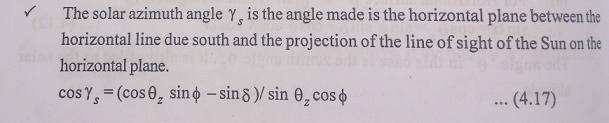## Chapter: Electric Energy Generation and Utilisation and Conservation : Solar Radiation and Solar Energy Collectors

In order to find the beam energy falling on a surface having any orientation, it is necessary to convert the value of the beam flux coming from the direction of the sun to an equivalent value corresponding to the normal direction to the surface.

In order to find the beam energy falling on a surface having any orientation, it is necessary to convert the value of the beam flux coming from the direction of the sun to an equivalent value corresponding to the normal direction to the surface.

If ŌĆśqŌĆÖ is the angle between an incident beam of flux ŌĆśIbnŌĆÖ and the normal to a plane surface, then the equivalent flux falling normal to the surface is given by IbnCosq. The angle ŌĆśqŌĆÖ can be related by a general equation to ŌĆśŽĢŌĆÖ latitude , ŌĆś╬▓ŌĆÖ the slope ŌĆś╬│ŌĆÖ the surface azimuth angle, ŌĆś╬┤ŌĆÖ the declination and ŌĆśŽēŌĆÖ the hour angle.

The Latitude ŌĆśŽĢŌĆÖ of a location is the angle made by the radial line joining the location to the centre of the earth with the projection of the line on the equatorial plane. It varies from ŌĆō 90o to +90o. By convention, the latitude is measured as positive for the northern hemisphere.

The slope ŌĆś╬▓ŌĆÖ is the angle made by the plane surface with the horizontal. It varies from 0 to 180o.

The surface Azimuth Angle ŌĆś╬│ŌĆÖ is the angle made in the horizontal plane between the horizontal line due south and the projection of the normal to the surface on the horizontal plane. It varies from -180o to + 180o.

We adopt the convention that the angle is positive, if the normal is East of South and Negative if West of South.

The Declination ŌĆś╬┤ŌĆÖ is the angle made by the line joining the centres of the Sun and the Earth with the projection of this line on the equatorial plane.Where, n is the day of the year(or Day Number).

The hour angle ŌĆśŽēŌĆÖ is an angular measure of time. It varies from -90o to +90o.

It is the angle through which the earth must be rotated to bring the meridian of plane directly under the sun. In order words, it is the angular displacement of sun east and west of the local meridian, due to the rotation of the earth on its axis 15o per hour. The hour angle is zero at solar noon, negative in the morning and positive in the afternoon(Table 4.2) for northern hemisphere and vice-versa for southern hemisphere. Expression for hour angle by Žē= (ST-12) 15o.Study Material, Lecturing Notes, Assignment, Reference, Wiki description explanation, brief detail
Electric Energy Generation and Utilisation and Conservation : Solar Radiation and Solar Energy Collectors : Solar Radiaion Geometry |